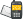It is currently 30 Oct 2020, 05:16### GMAT Club Daily Prep

#### Thank you for using the timer - this advanced tool can estimate your performance and suggest more practice questions. We have subscribed you to Daily Prep Questions via email.

Customized
for You

we will pick new questions that match your level based on your Timer History

Track

every week, we’ll send you an estimated GMAT score based on your performance

Practice
Pays

we will pick new questions that match your level based on your Timer History

#### Not interested in getting valuable practice questions and articles delivered to your email? No problem, unsubscribe here.# Probability that at least one of the event occurs is ?Question banks Downloads My Bookmarks Reviews Important topics
Author Message
TAGS:InternJoined: 20 Mar 2018
Posts: 39
GRE 1: Q163 V149GRE 2: Q168 V162GPA: 3.5
Followers: 1

Kudos [?]: 40  , given: 8

Re: Probability that at least one of the event occurs is ? [#permalink]
1
KUDOS
https://greprepclub.com/forum/qotd-19-f ... tml#p18435
A similar question with official explanation
ManagerJoined: 26 Jan 2018
Posts: 189
GRE 1: Q165 V156Followers: 1

Kudos [?]: 135 , given: 3

Re: Probability that at least one of the event occurs is ? [#permalink]
Highest is atleast one of them happening, hence 0.2 or 0.3

Other option is when both occur 0.2*0.3 = 0.06

Hence the range is 0.06 to 0.3.

InternJoined: 19 Mar 2018
Posts: 14
Followers: 0

Kudos [?]: 9 , given: 4

Re: Probability that at least one of the event occurs is ? [#permalink]
novice07 wrote:
https://greprepclub.com/forum/qotd-19-for-a-certain-probability-experiment-the-probabil-2639.html#p18435
A similar question with official explanation

Thanks a lot. That solution is perfect.

Posted from my mobile deviceManagerJoined: 26 Jan 2018
Posts: 189
GRE 1: Q165 V156Followers: 1

Kudos [?]: 135  , given: 3

Re: Probability that at least one of the event occurs is ? [#permalink]
1
KUDOS
Either of the event occur, 0.2 or 0.3,

Both events occur = 0.2*0.3
=0.06

So the range is 0.06 to 0.3InternJoined: 05 Mar 2018
Posts: 3
Followers: 0

Kudos [?]: 1  , given: 4

Re: Probability that at least one of the event occurs is ? [#permalink]
1
KUDOS
0.44
P( at least one event occur ) = 1-P(no event occurring )

P( no event occurring) = [1-P(A)] *[1-P(B)] =(1-0.2)*(1-0.3) =0.8*0.7=0.56

Hence P( at least one event occur ) = 1-0.56 =0.44

InternJoined: 14 Apr 2018
Posts: 7
Followers: 0

Kudos [?]: 5 , given: 5

Re: Probability that at least one of the event occurs is ? [#permalink]
novice07 wrote:
Peter wrote:
novice07 wrote:
If P(A) = 0.8 and P(B) = 0.7, then Events A and B have to be independent for P(A and B) to be 0. Since they are not (which we assume when not given otherwise) there must be some intersection of these events.
So, if P(A) is 0.8 and P(B) is 0.7 then P(A and B)maximum would be 1, since it can't exceed 1.

Your concept is weak. For independent events, events are said not to affect each other, but they can both occur.

For example, the tail of a coin can occur with a dice rolling 6.

however, mutually exclusively events would not allow both events to occur, for example the tail and the face of a coin can not both occur.

Also the it is the maximum of (A or B) NOT (A and B) is 1. you should draw 2 circles which one stands for P(A) 0.8, the other stands for P(B) 0.7. Then the maximum of (A and B) is 0.7 when P(B) 0.7 is included in P(A) 0.8.

That maximum of P(A or B) NOT (A and B) was an overlook. Sorry for that.

I know that independent events can occur together and in that case P(A and B) would be P(A)*P(B). Appreciate for the example. However, I just meant that P(A or B) can't be equal to 1.5 because if two events having probabilities 0.7 and 0.8, it is not possible to have zero intersection.

Hi guys!!
Do you know what is the correct answer for this question?
If we understand "Probability that at least one of the event occurs is" as "The probability that A or B or Both will occur?", the correct answer should be 0.3 to 0.5, right?
ManagerJoined: 26 Jan 2018
Posts: 189
GRE 1: Q165 V156Followers: 1

Kudos [?]: 135 , given: 3

Re: Probability that at least one of the event occurs is ? [#permalink]
cyanApples wrote:
So if P(A) = 0.8 and P(B) = 0.7 then max probability is 1.5 ? That doesnt seem right.

Posted from my mobile deviceImportant thing to be noted. Probability of any event happening ranges between 0 and 1. Probability cannot have value beyond this range.Re: Probability that at least one of the event occurs is ?   [#permalink] 11 May 2018, 04:20
Display posts from previous: Sort by

# Probability that at least one of the event occurs is ?Question banks Downloads My Bookmarks Reviews Important topics
 Go to page   Previous    1   2   [ 27 posts ]Powered by phpBB © phpBB Group Kindly note that the GRE® test is a registered trademark of the Educational Testing Service®, and this site has neither been reviewed nor endorsed by ETS®.